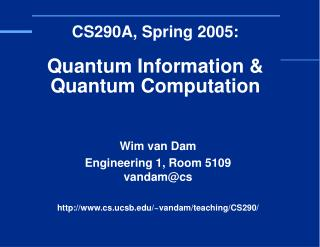# CS290A, Spring 2005: Quantum Information & Quantum Computation - PowerPoint PPT PresentationDownload PresentationCS290A, Spring 2005: Quantum Information & Quantum Computation

CS290A, Spring 2005: Quantum Information & Quantum Computation
Download Presentation## CS290A, Spring 2005: Quantum Information & Quantum Computation

- - - - - - - - - - - - - - - - - - - - - - - - - - - E N D - - - - - - - - - - - - - - - - - - - - - - - - - - -
##### Presentation Transcript

1. Wim van Dam Engineering 1, Room 5109vandam@cs http://www.cs.ucsb.edu/~vandam/teaching/CS290/ CS290A, Spring 2005:Quantum Information & Quantum Computation

2. Administrivia • Exercises have been posted.Try to solve them, get help if you have problems • Questions about the questions? • Other questions?

3. Efficient Quantum Circuits |0 |1 |0 |ψoutput? |1 |0 - Start with n classical bits as input.- Apply a sequence of poly(n) elementary gates- Measure the outcome ψoutput.

4. This Week • Mathematics of Quantum Mechanics: • Braket calculus. • Finite dimensional unitary transformations; eigenvector/eigenvalue decompositions. • Projection Operators. • Circuit Model of Quantum Computation: • Examples of important gates. • Composing quantum gates into quantum circuits. • (Classical) Reversible computation. • Universality results for quantum circuits.

5. Hermitian Conjugates • See handout “Mathematics of Quantum Computation” • Generalization of complex conjugate* to matrices. • Procedure: “Flip & conjugate” • Notation: |ψ† = ψ| for vectors and M† for matrices:

6. Inner / Outer Products • |x is a column vector, x| is a row vector. • Inner Product x|y gives a -valued scalar • Outer product |yx| gives a DD -valued matrix: Notation: |rc| with r,c{1,…,D} denotes the 0-matrix,with a “1” in the r-th row and c-th column.Hence for matrices M = ij Mij|ij| and M† = ij M*ji|ij|

7. Products of Bras and Kets • How to deal with product sequences? • Leave out the bars and dots: ψ|·|φ = ψ|φ • They don’t commute: φ|ψ≠ψ|φ • Keep on eye on the dimensions: |ψ is a vector, ψ|ψ a scalar and |ψψ| is a matrix. • They are distributive and associative:φ|(α|ψ+β|ψ’) = αφ|ψ+βφ|ψ’(|ψφ|)(|φψ|) = |ψ(φ|φ)ψ| = |ψψ|

8. Preserving Norms • The norm of a vector α|v+β|w, is determined by:║α|v+β|w║2 = (α*v|+β*w|)(α|v+β|w) = α*αv|v + β*βw|w + α*βv|w + β*αw|v = α*α + β*β + 2Real(α*βv|w) • Two vectors |v, |w are mutually orthogonal, if and only if v|w = 0; in which case ║α|v+β|w║2 = |α|2+|β|2. • If T is a linear, norm preserving transformation of |v,|w, then the inner product between (T|v)† and T|w has to be the same as v|w. Hence: T has to be inner product preserving.

9. Unitarity 1 • Let M be a linear, norm preserving (= unitary) D-dimensional transformation on the Hilbert space D. • When represented as a DD -valued matrix, how do we determine that M is unitary? • Because M|1, M|2,…, M|D have to have norm one,the columns of M have to have norm one. • Because |1, |2,…, |D are mutually orthogonal,the columns of M have to be mutually orthogonal.

10. Unitarity 2 • Let MDD be the matrix of a unitary transformation. • The columns M|1, M|2,…, M|D have to form a D-dimensional orthonormal basis, hence M†·M = I: • M is invertible: M-1 = M†, which is also unitary. • The identity matrix is unitary • The set of D-dimensional unitary transformations is a (matrix) group.

11. Recognizing Unitarity • Perform the matrix multiplication: M†·M = M·M† = I?Simple for small matrices, impractical for larger ones. • Prove that M|1,…, M|D are mutually orthogonal. • If M is a classical computation, then the above means that M|1,…, M|D has to be a permutation.Alternatively, a classical M has to be reversible. • Topic of (classical) reversible computation.

12. Reversible Computation • Standard computation is irreversible: (a,b)  (a AND b) • Reversible gates have FAN-IN = FAN-OUT. • Irreversible gates: (a,b)  (a OR b), (a) (0), but also: (a,b)  (a, a OR b) • Reversible gates: (a) (~a), CNOT:(a,b)  (a, ba), CCNOT:(a,b,c)  (a,b,cab), and C-SWAP:

13. Reversibility Issues For general F:{0,1}n{0,1}n|x  |F(x) is irreversible |F(x) |x F For reversible F:{0,1}n{0,1}n|x  |F(x) is reversible |F(x) |x F For general F:{0,1}n{0,1}n|x,y  |x,yF(x) is reversible |x,y |x,yF(x) Id,F Which reversible functions can we implement efficiently under the assumption that we can implement F efficiently?

14. CC-NOTs as Universal Gates • With CCNot gates, we can implement NOT and AND:CCNOT:|1,1,c  |1,1,~c, CCNOT:|a,b,0  |a,b,ab. • If we keep old memory around, any circuit function Fcan be implemented efficiently |x,0,0  |x,gx,F(x) • By copying the output F(x) and running the circuit in reverse, we can erase the garbage bits gx: |x,gx,F(x),0  |x,gx,F(x),F(x)  |x,0,0,F(x). • In sum: |x,0,0  |x,F(x),0 can be implemented efficiently as long as we have clean 0-qubits around.

15. Power of Reversible Computation • We showed that the requirement of reversibility does not change (significantly) the efficiency of our computations: Reversible Computation = General Computation. • But what about the efficiency of implementing of other reversible computations?

16. Problematic Reversibility • If F is a reversible function (a permutation of {0,1}n),then |x  |F(x) is reversible. • Even if F can be implemented efficiently (classically),it does not always hold that |x  |F(x) can be implemented in a unitary/reversible way. • |x,0  |x,F(x) can be done efficiently, but |x,F(x)  |0,F(x) can be hard. • Reason: F-1 may be hard to implement (one-way F).

17. More on Reversibility • Reversibility also plays a role in the heat production of bit operations: kBT ln(2) ~ 10–22 Joule per bit. • Remember: A Quantum Computation can always just as easily be done in reverse:Just read the circuit right from left, and invert each unitary gate along the way. • See in “Quantum Computation and Quantum Information”: §3.2.5, “Energy and Computation”